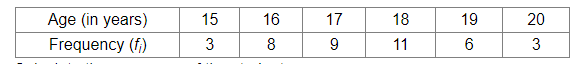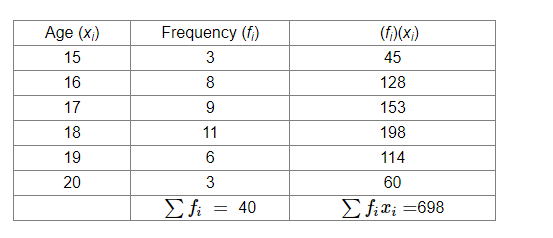# The following data give the number of boys of a particular age in a class of 40 students.Question:

The following data give the number of boys of a particular age in a class of 40 students.Calculate the mean age of the students.

Solution:

We will make the following table:Thus, we have:

Mean $=\frac{\sum f_{i} x_{i}}{\sum x_{i}}$

$=\frac{698}{40}=17.45$ years